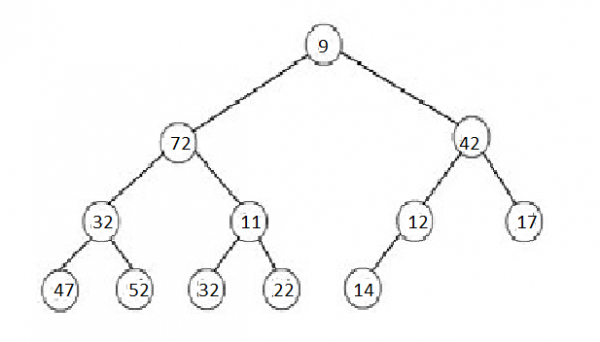# Min-Max Heaps

A min-max heap is defined as a complete binary tree containing alternating min (or even) and max (or odd) levels. Even levels are denoted as for example 0, 2, 4, etc, and odd levels are denoted as 1, 3, 5, etc.

We consider in the next points that the root element is at the first level, i.e., 0.Example of Min-max heap

## Features of Min-max heap

• Each node in a min-max heap is associated with a data member (usually called key) whose value is implemented to calculate the order of the node in the min-max heap.
• The root element is the minimum element in the min-max heap.
• One of the two elements in the second level, which is a max (or odd) level, is the maximum element in the min-max heap
• Let y be any node in a min-max heap.
• If y is on a min (or even) level, then y.key is the smallest key among all keys in the subtree with root y.
• If y is on a max (or odd) level, then y.key is the highest key among all keys in the subtree with root y.
• A node on a min (max) level is denoted as a min (max) node.

A max-min heap is defined as opposite to min-max heap; in such a heap, the highest value is stored at the root, and the minimum value is stored at one of the root's children.